# Fitting the COMIX algorithm to multi-sample data

In the following, we provide an application-oriented overview of COMIX and its main parameters through a demonstration of the COMIX code and its various utility and diagnostic functions. The presentation will provide guidelines for choosing the main parameters of interest and assessing the convergence of the MCMC chain.

We start by fitting the model to data that are simulated from 5 clusters in 3 dimensions that are not aligned across 4 samples. The clusters are drawn from a multivariate skew normal distribution.

# Number of clusters:
K <- 5
k_names <- paste0("Cluster", 1:K)
# Number of samples:
J <- 4
j_names <- paste0("Sample", 1:J)
# Dimension:
p <- 3
p_names <- paste0("p", 1:p)
# For the sake of the demonstration, we will generate three large clusters (1, 2 and 3)
# and two smaller clusters (4 and 5):

n_jk <-
matrix(
c(
200, 210, 290, 30, 40,     # First row for the five clusters of the first sample
220, 190, 340,  0, 50,     # Second row for the five clusters of the second sample
0, 180, 340, 60, 60,     # Third row for the five clusters of the third sample
150, 240, 310, 50, 40      # Fourth row for the five clusters of the fourth sample
),
byrow = TRUE,
nrow = 4,                    # Number of samples
ncol = 5,                    # Number of clusters
dimnames = list(j_names, k_names)
)

print(n_jk)
##         Cluster1 Cluster2 Cluster3 Cluster4 Cluster5
## Sample1      200      210      290       30       40
## Sample2      220      190      340        0       50
## Sample3        0      180      340       60       60
## Sample4      150      240      310       50       40

Note that the first cluster is absent from the third sample and the fourth cluster absent from the second sample.

Thus, the total number of observations is:

(n <- sum(n_jk))
##  3000

Next, we sample parameters for the different clusters and samples in a fashion similar to that of the generative model of COMIX. The model assumes that cluster locations for each sample are drawn from a multivariate normal distribution around a “grand” cluster location. We denote this parameter as $$\xi_{0,k}$$, a single $$\xi_0$$ parameter for each cluster $$k$$. Here, there are 5 grand cluster locations, $$\xi_{0,1},...,\xi_{0,5}$$, one for each cluster. These too are drawn from a multivariate normal distribution, which we center at $$b_0=(0,0,0)$$ and set the variance-covariance matrix $$B_0$$, set to be $$8\cdot I$$:

set.seed(1)
b0 <- rep(0, p)
B0 <- 8 * diag(p)
xi0 <- t(MASS::mvrnorm(K, b0, B0))
colnames(xi0) <- paste0("xi0,", 1:K)
rownames(xi0) <- p_names

print(xi0)
##        xi0,1     xi0,2     xi0,3     xi0,4      xi0,5
## p1  4.275963 1.1026432 -1.757134 -6.264117  3.1817851
## p2 -2.320635 1.3786576  2.088298  1.628556 -0.8637688
## p3 -1.771879 0.5194218 -2.363515  4.512135  0.9319887

xi0 is a $$3\times5$$ matrix, where each of the 5 columns for the 5 clusters is a point in a 3-dimensional space.

The variance-covariance matrix, that alongside $$\xi0$$ paramaterizes the multivariate normal distribution of cluster locations around the grand locations, denoted by $$E_k$$ (one for each of the 5 clusters) determines the amount of misalignment between clusters. For the sake of the example, we’ll set clusters 2 and 5 to have larger misalignment, and clusters 1, 3 and 4 to be very nearly aligned. This can be done in the following manner:

E <- list()

# The 3 well aligned clusters:
E[] <- 0.001 * diag(p)
E[] <- 0.001 * diag(p)
E[] <- 0.001 * diag(p)

# The 2 misaligned clusters:
E[] <- 0.25 * diag(p)
E[] <- 0.25 * diag(p)

names(E) <- paste0("E", 1:K)
E <- lapply(E, function(e) {rownames(e) <- p_names; colnames(e) <- p_names; e})

So, for example, the variance-covariance matrix for the first cluster is:

print(E)
## $E1 ## p1 p2 p3 ## p1 0.001 0.000 0.000 ## p2 0.000 0.001 0.000 ## p3 0.000 0.000 0.001 Based on the parameters $$\xi_{0,k}$$ and E_k we can draw location parameters $$\xi_{j,k}$$ for each sample and cluster (every element of the following list corresponds to a single cluster with 4 values of xi of dimension 3): xi <- NULL for (k in 1:K) { xi <- bind_rows( xi, bind_cols( expand_grid(Sample = 1:J, Cluster = k), as_tibble(MASS::mvrnorm(J, xi0[ , k], E[[k]])) ) ) } And so we can see, for example, that the first cluster has location parameters that are nearly equal across all samples: xi %>% filter(Cluster == 1) ## # A tibble: 4 × 5 ## Sample Cluster p1 p2 p3 ## <int> <int> <dbl> <dbl> <dbl> ## 1 1 1 4.21 -2.30 -1.77 ## 2 2 1 4.30 -2.29 -1.77 ## 3 3 1 4.27 -2.30 -1.74 ## 4 4 1 4.27 -2.32 -1.75 And the second cluster has location parameters that differ much more between samples: xi %>% filter(Cluster == 2) ## # A tibble: 4 × 5 ## Sample Cluster p1 p2 p3 ## <int> <int> <dbl> <dbl> <dbl> ## 1 1 2 0.895 1.33 -0.216 ## 2 2 2 0.905 1.57 0.280 ## 3 3 2 1.07 1.35 0.728 ## 4 4 2 1.65 0.690 1.20 In addition to a location parameter, each multivariate skew normal cluster is also paramaterized with a scale $$p\times p$$ matrix parameter $$\Sigma_k$$, and a skewness vector $$\alpha_k$$ of length $$p$$. Let’s choose some random scale parameters for the different clusters: Sigma <- list() for (k in 1:K) { Sigma[[k]] <- matrix(0.1, p, p) + diag(0.2, p, p) tmp <- rep(0, p) tmp[sample(1:p, 1)] <- 0.1 Sigma[[k]] <- Sigma[[k]] + diag(tmp) } Set clusters 1 and 2 and 5 with no skewness, and clusters 3 and 4 with heavy skewness in one of the margins: alpha <- matrix(0, nrow = p, ncol = K, dimnames = list(p_names, k_names)) # First margin of the skewness vector of third cluster: alpha[1, 3] <- 5 # Second margin of the skewness vector of fourth cluster: alpha[2, 4] <- -6 print(alpha) ## Cluster1 Cluster2 Cluster3 Cluster4 Cluster5 ## p1 0 0 5 0 0 ## p2 0 0 0 -6 0 ## p3 0 0 0 0 0 The multivariate skew normal distribution has an alternative parameterization, where the pair $$(\psi, G)$$ substitutes the pair $$(\alpha, \Sigma)$$. The MCMC chain estimates the latter pair. Let us use the utility function transform_params to compute the 5 alternative pairs (which we will later use to evaluate convergence): psi_G <- list() for (k in 1:K) { psi_G[[k]] <- COMIX::transform_params(Sigma[[k]], alpha[ , k]) } Note that when $$\alpha_k = 0_p$$, $$\psi_k$$ is also $$0_p$$, in which case $$G_k=\Sigma_k$$: psi_G[]$G
##      [,1] [,2] [,3]
## [1,]  0.3  0.1  0.1
## [2,]  0.1  0.4  0.1
## [3,]  0.1  0.1  0.3
Sigma[]
##      [,1] [,2] [,3]
## [1,]  0.3  0.1  0.1
## [2,]  0.1  0.4  0.1
## [3,]  0.1  0.1  0.3

But when $$\alpha_k(i) \ne 0$$ for some $$i$$, both parameters differ:

psi_G[]$psi ## [,1] ## [1,] 0.5370862 ## [2,] 0.1790287 ## [3,] 0.1790287 alpha[ , 3] ## p1 p2 p3 ## 5 0 0 psi_G[]$G
##             [,1]        [,2]        [,3]
## [1,] 0.011538462 0.003846154 0.003846154
## [2,] 0.003846154 0.267948718 0.067948718
## [3,] 0.003846154 0.067948718 0.367948718
Sigma[]
##      [,1] [,2] [,3]
## [1,]  0.3  0.1  0.1
## [2,]  0.1  0.3  0.1
## [3,]  0.1  0.1  0.4

We are now ready to sample the 3000 multivariate skew normal data points into a $$3000\times p$$ matrix Y, and in addition generate an integer vector C of length 3000, denoting for each row of Y the sample from which the corresponding observation is drawn. As a sanity check, we also denote for each observation which cluster it was drawn from in a vector of length 3000 named t:

Y <- NULL
C <- NULL
t <- NULL
for (j in 1:J) {
for (k in 1:K) {
xi_jk <- xi %>% filter(Sample == j, Cluster == k) %>% select(all_of(p_names)) %>% unlist()
Y <-
rbind(
Y,
sn::rmsn(n_jk[j, k], xi = xi_jk, Sigma[[k]], alpha = alpha[ , k])
)
C <- c(C, rep(j, n_jk[j, k]))
t <- c(t, rep(k, n_jk[j, k]))
}
}

# Sanity check:
print(table(C, t))
##    t
## C     1   2   3   4   5
##   1 200 210 290  30  40
##   2 220 190 340   0  50
##   3   0 180 340  60  60
##   4 150 240 310  50  40
print(n_jk)
##         Cluster1 Cluster2 Cluster3 Cluster4 Cluster5
## Sample1      200      210      290       30       40
## Sample2      220      190      340        0       50
## Sample3        0      180      340       60       60
## Sample4      150      240      310       50       40
print(all(table(C, t) == n_jk))
##  TRUE
# Make tibbles and naming variables for plotting:
Y_tb <- Y
colnames(Y_tb) <- p_names
Y_tb <-
bind_cols(
tibble(Sample = factor(C), Cluster = factor(t)),
as_tibble(Y_tb)
)

xi0_tb <- as_tibble(t(xi0)) %>% mutate(Cluster = factor(1:K))

Cluster_names <- paste("Cluster", 1:K)
names(Cluster_names) <- 1:K

Sample_names <- paste("Sample", 1:J)
names(Sample_names) <- 1:J

Let’s get a sense of the data - start by looking at all Clusters and samples together:

ggplot(Y_tb) +
geom_point(aes(x = p1, y = p2, col = Cluster, shape = Sample), alpha = 0.7) +
theme_bw()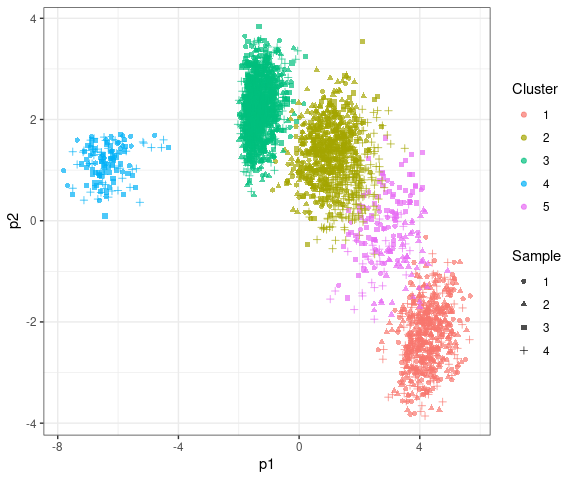ggplot(Y_tb) +
geom_point(aes(x = p2, y = p3, col = Cluster, shape = Sample), alpha = 0.7) +
theme_bw()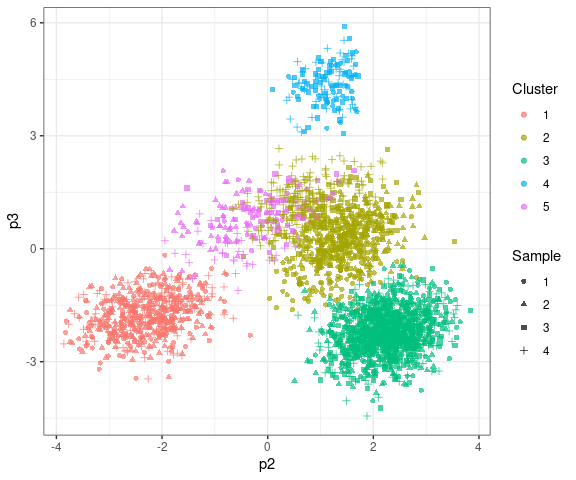And then facet to show each cluster and sample separately.

$$p_1$$-$$p_2$$ margins:

ggplot(Y_tb) +
geom_point(aes(x = p1, y = p2, col = Cluster), alpha = 0.25) +
facet_grid(
Sample ~ Cluster,
labeller = labeller(Cluster = Cluster_names, Sample = Sample_names)
) +
geom_hline(data = xi0_tb, aes(yintercept = p2, col = Cluster)) +
geom_vline(data = xi0_tb, aes(xintercept = p1, col = Cluster)) +
geom_point(data = xi0_tb, aes(x = p1, y = p2), alpha = 0.7) +
xlab(expression(p)) +
ylab(expression(p)) +
theme_bw() +
theme(legend.position = "none")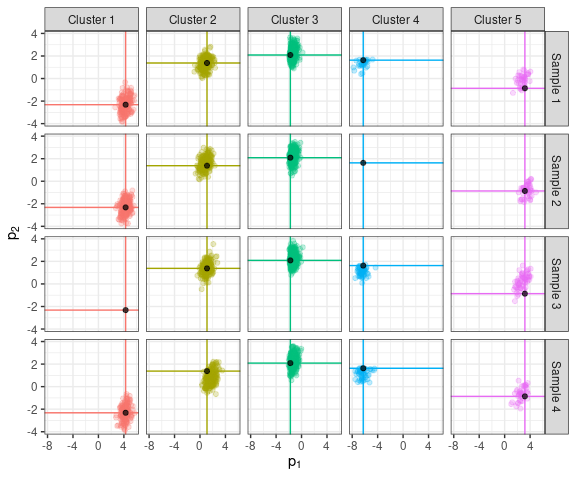$$p_2$$-$$p_3$$ margins:

ggplot(Y_tb) +
geom_point(aes(x = p2, y = p3, col = Cluster), alpha = 0.25) +
facet_grid(
Sample ~ Cluster,
labeller = labeller(Cluster = Cluster_names, Sample = Sample_names)
) +
geom_hline(data = xi0_tb, aes(yintercept = p3, col = Cluster)) +
geom_vline(data = xi0_tb, aes(xintercept = p2, col = Cluster)) +
geom_point(data = xi0_tb, aes(x = p2, y = p3), alpha = 0.7) +
xlab(expression(p)) +
ylab(expression(p)) +
theme_bw() +
theme(legend.position = "none")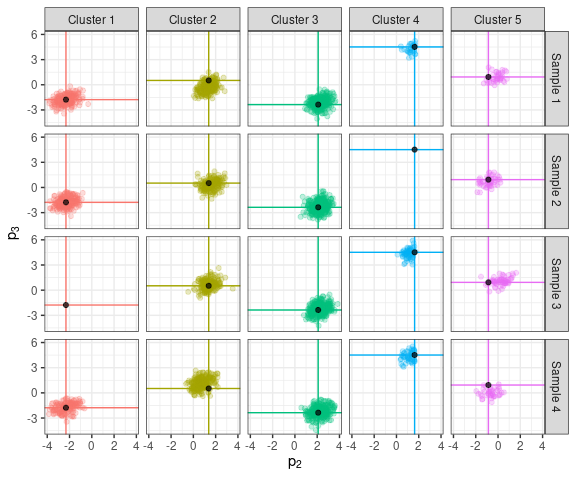We can see that clusters 1, 3, and 4 are aligned well, whereas clusters 2 and 5 are misaligned, as can be assessed from the deviation of the clusters from their grand locations (black dots).

## Fitting the model

The maximal number of clusters, $$K$$, should be chosen to exceed the expected number of clusters in the data. Since the algorithm is expected to merge clusters during the first iterations, we recommend setting a burn-in period of at least several hundreds iterations.

And so, a reasonable attempt at a first fit for our data could be:

set.seed(1)
prior <- list(zeta = 1, K = 10)

Where $$\zeta$$ is a coarsening parameter (to be discussed in a following section) and $$K$$ the maximal number of clusters.

pmc <- list(npart = 10, nburn = 250, nsave = 250)

npart is the number of particles in the Population Monte Carlo chain. More particles increase the accuracy of the estimated parameters, at the cost of more computations (and memory requirements). 10 is a reasonable amount to start with, which can be chosen larger based on post-hoc analyses of the initial setting.

Fit the model:

res <- comix(Y, C, pmc = pmc, prior = prior)
## initializing all particles...
## Done
## Merged clusters (iteration 5)
## Merged clusters (iteration 8)
## Merged clusters (iteration 17)
## Merged clusters (iteration 42)
## Iteration: 100 of 500
## Iteration: 200 of 500
## Iteration: 300 of 500
## Iteration: 400 of 500
## Iteration: 500 of 500

Since the MCMC chain may result with spurious label changes, it is recommended to run the function relabelChain() on the output to reduce potential problems:

resRelab <- relabelChain(res)
## K=10
## T=250
## N=3000
## J=4
## p=3

The res and resRelab objects contain the full MCMC chains. To compute posterior point estimates for the different parameters, use:

res_summary <- summarizeChain(resRelab)

Then, for instance, we can tabulate the estimated cluster labels by sample:

t(table(res_summary$t, C)) ## ## C 1 2 4 5 6 ## 1 210 30 200 40 290 ## 2 190 0 219 51 340 ## 3 182 60 0 58 340 ## 4 240 50 150 40 310 Since we know the true cluster labels we can compare the estimates to those: n_jk ## Cluster1 Cluster2 Cluster3 Cluster4 Cluster5 ## Sample1 200 210 290 30 40 ## Sample2 220 190 340 0 50 ## Sample3 0 180 340 60 60 ## Sample4 150 240 310 50 40 Indeed, the classification has matched nearly perfectly the true labels. Next, we can calibrate the data given the model fit: calibrated_data <- calibrate(resRelab) (for very large data sets, consider using calibrateNoDist() that stores less information) And plot the calibrated results (with the estimated cluster labels) in comparison to the original data: Y_cal <- calibrated_data$Y_cal
colnames(Y_cal) <- paste0("p", 1:p, "_cal")
Y_tb <-
bind_cols(
Y_tb,
as_tibble(Y_cal)
)
Y_tb$Estimated_Cluster <- as.character(res_summary$t)

True_cluster_names <- paste("True Cluster", 1:K)
names(True_cluster_names) <- 1:K

non_trivial_clusters <- unique(Y_tb$Estimated_Cluster) # Post-hoc manual mapping of estimated cluster labels # to true cluster labels based on estimated sizes: True_vs_Estimated_Cluster_Names <- c(1, 2, 3, 4, 5) # (adjust the above line when running code for different model fits) Estimated_Cluster_Labels <- as.integer(non_trivial_clusters) estimated_xi0 <- t(res_summary$xi0[ , Estimated_Cluster_Labels])
colnames(estimated_xi0) <- paste0("p", 1:p, "_cal")
estimated_xi0_tb <-
tibble(
as_tibble(estimated_xi0),
Estimated_Cluster = factor(Estimated_Cluster_Labels),
Cluster = factor(True_vs_Estimated_Cluster_Names)
)

ggplot(Y_tb) +
geom_point(aes(x = p1_cal, y = p2_cal, col = Estimated_Cluster), alpha = 0.35) +
facet_grid(
Sample ~ Cluster,
labeller = labeller(Cluster = True_cluster_names, Sample = Sample_names)
) +
geom_hline(data = estimated_xi0_tb, aes(yintercept = p2_cal), col = "black", alpha = 0.25) +
geom_vline(data = estimated_xi0_tb, aes(xintercept = p1_cal), col = "black", alpha = 0.25) +
geom_point(data = estimated_xi0_tb, aes(x = p1_cal, y = p2_cal), alpha = 0.7) +
xlab(expression(p)) +
ylab(expression(p)) +
theme_bw() +
theme(legend.position = "none")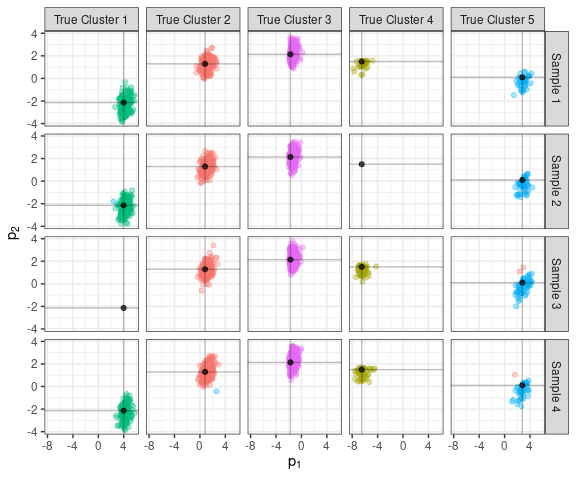In the above plot the calibrated data are plotted. The intersection of the grey lines at the black circle denotes the estimated grand location cluster parameter. The columns are faceted by true cluster labels and the color coding corresponds to the estimated cluster labels. We can see the few points that were classified wrongly in Sample 4, True Cluster 2 and Samples 3 and 4, True Cluster 5. The calibrated data are indeed much better aligned in comparison to the original data.

## MCMC Diagnostics

The COMIX package includes several diagnostic functions to assess convergence of the MCMC chain.

• effectiveSampleSize() computes the effective sample sizes for each of the model parameters (based on the effectiveSize() function from the coda package), and plotEffectiveSampleSize() offers one possible visualization (different for each of the model parameters) to explore the results.

• plotTracePlots() generates trace plots for each of the model parameters.

• heidelParams() computes a test of stationarity of the MCMC chain, as well as a criterion of relative accuracy for the estimate of the mean (based on the heidel.diag() function from the coda package). plotHeidelParams() offers a visualuzation for these results.

• gewekeParams() computes a test for convergence of chains based on a test for equality of the means of the first and last part of a chain (based on the geweke.diag() function form the coda package). plotGewekeParams() offers a visualuzation for these results.

• acfParams() generates auto-correlation plots for the various parameters of the model (based on the acf() function).

The functions effectiveSampleSize(), plotTracePlots(), heidelParams(), gewekeParams() and acfParams() take as input either an object of class COMIX (a model fit or a relabeled model fit) or an object of class tidyChainCOMIX, resulting from tidyChain that applies to a COMIX object. In order to avoid running tidyChain() multiple times in the background, we first apply it to the relabeled results, store the output and use it as input for the diagnostic functions.

tidy_chain <- tidyChain(resRelab)

Although the clusters are more intuitively parameterized by $$\Sigma$$, a scale matrix, and $$\alpha$$, a vector for skewness, the sampler generates posterior draws for the $$G$$ matrix and $$\psi$$ vector, which jointly provide an alternative parameterization for $$\Sigma$$ and $$\alpha$$. After the posterior estimates for $$G$$ and $$\psi$$ are computed, $$\Sigma$$ and $$\alpha$$ are computed from those. However, in order to evaluate the posterior’s distribution behavior and to assess convergence of the chain, the diagnostic functions are applied to $$G$$ and $$\psi$$.

### Trace plots

In order to plot trace plots of the posterior parameter estimate samples, use the plotTracePlots() function. Trace plots can be shown for each of the following parameters: w, the cluster- and sample- specific weights (denoted in the manuscript by $$\omega_{j,k}$$), xi0 the grand location parameters E the variance-covariance matrix of the distribution from which the sample specific location parameters are drawn, xi the sample specific location parameters, psi and G, the parameters that jointly determine the scale and skewness of each of the clusters, and eta, a concentration parameter for the Griffiths-Engen-McCloskey (GEM) prior on the weights.

Show trace plots for the weights, where color coding corresponds to cluster (from 1 to $$K$$):

plotTracePlots(tidy_chain, "w")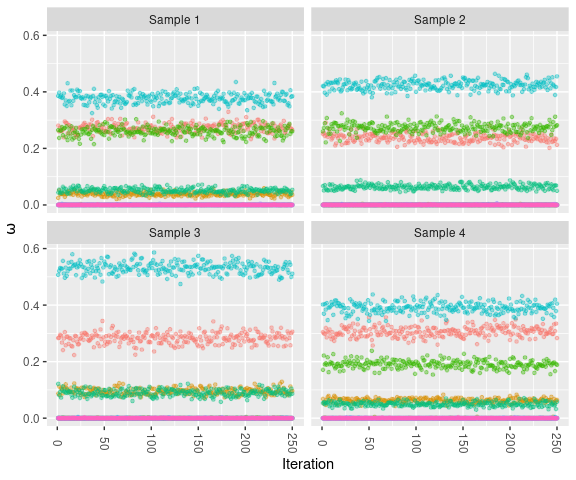Show trace plots for the grand location parameters, where color coding corresponds to margin (from 1 to $$p$$):

plotTracePlots(tidy_chain, "xi0")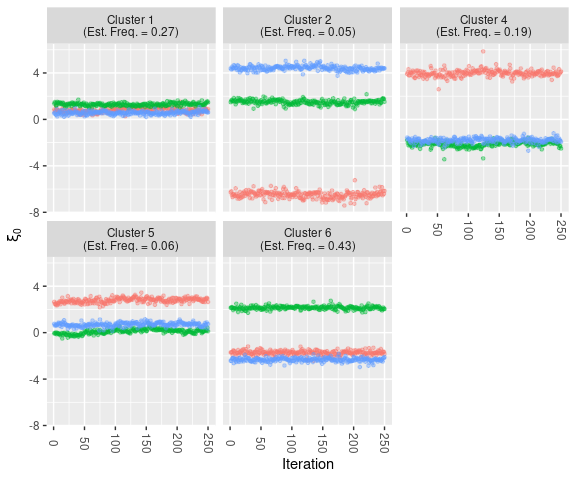Trace plots for the sample specific location parameters, where color coding corresponds to margin (from 1 to $$p$$):

plotTracePlots(tidy_chain, "xi")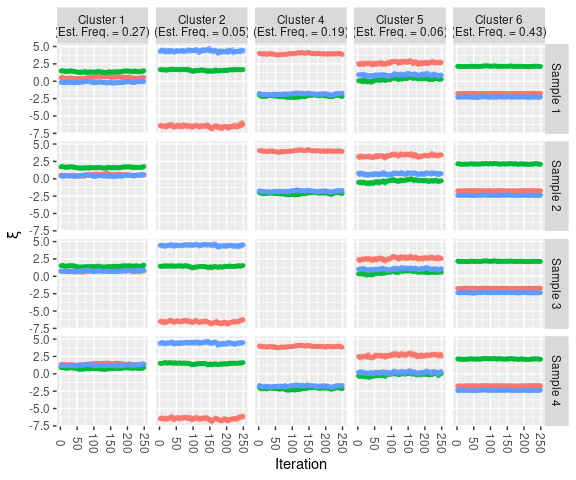Trace plots for the variance covariance matrix of the grand location parameters, where color coding corresponds to margin (from 1 to $$p$$):

plotTracePlots(tidy_chain, "E")